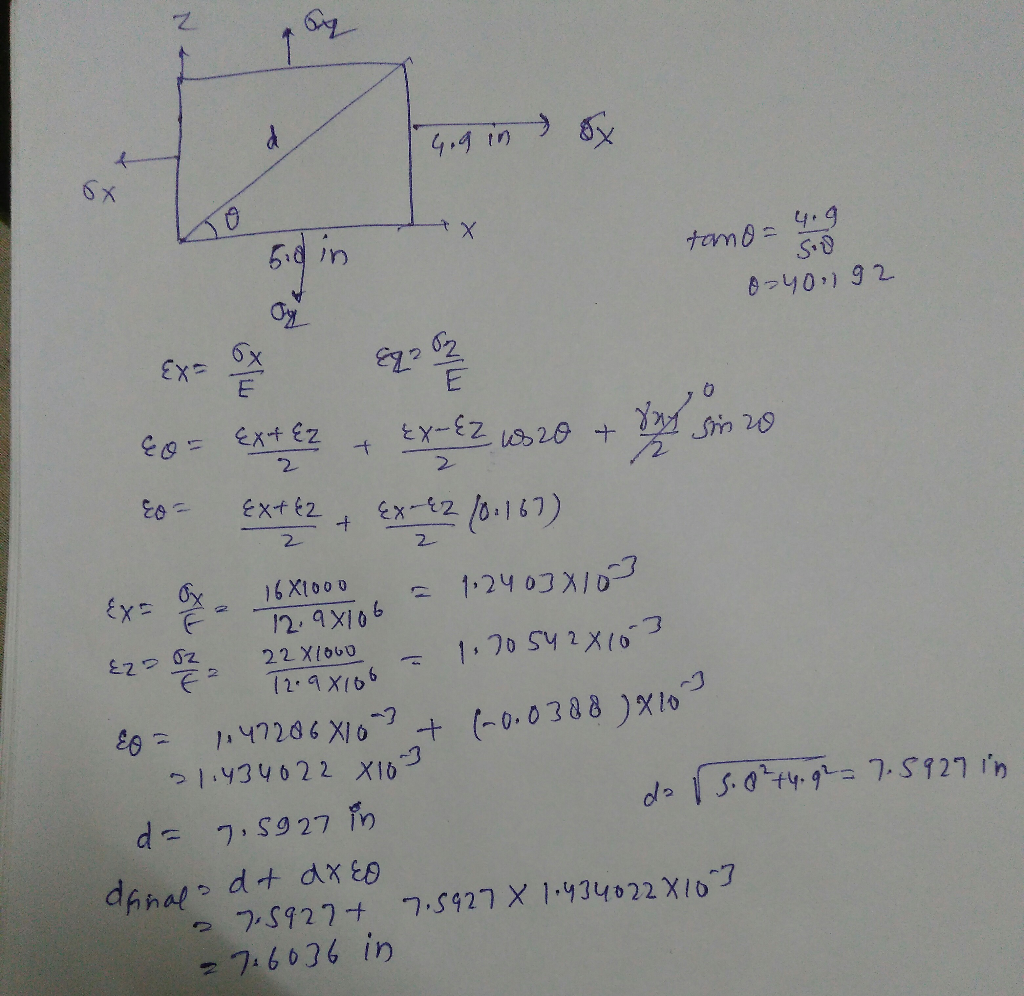# A piece of fabric used in a hot air-balloon is Lx = 5.8 in. long in...

A piece of fabric used in a hot air-balloon is Lx = 5.8 in. long in the x-direction and Lz = 4.9 in. long in the z-direction. The fabric is inflated and results in a biaxial loading that results in normal stresses \sigma_x σ x = 16 ksi and \sigma_z σ z = 22 ksi. Knowing that the properties of the fabric can be approximated as E = 12.9 × 10^6 psi and \upsilon υ = 0.33, determine the new length of the diagonal AC. The answer should be inches with 2-decimal accuracy.​​​​​​